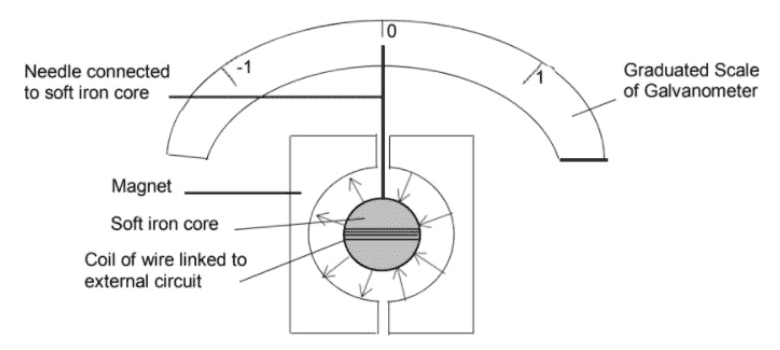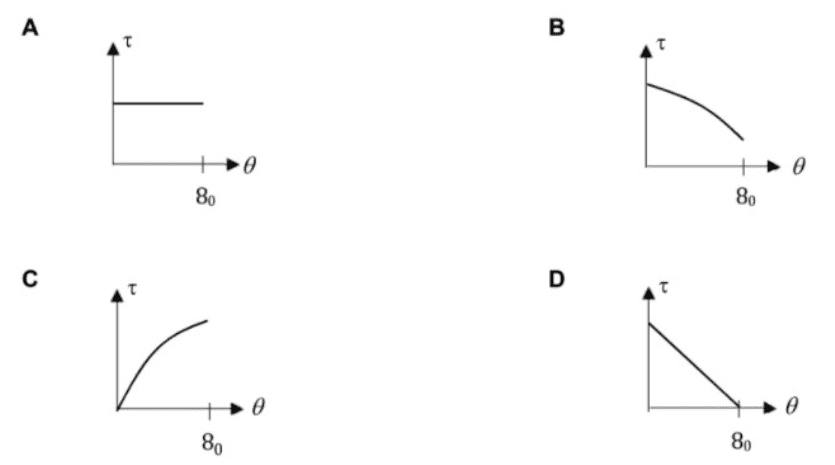# Graph of torque against displacement of galvanometer needle

• songoku

#### songoku

Homework Statement
The diagram below is a galvanometer. A coil is wound around a circular core which is placed between two magnets with circular surface such that the magnetic field (indicated by arrows) on the surface of iron core is directed perpendicularly onto the surface and of the same magnitude across the surface of iron core.

When a constant current flows, the needle will be deflected to angle ##8_0## from the vertical direction. Which of the following shows the variation of torque on soft iron core ##\tau## with angular displacement ##\theta##?
Relevant Equations
##\tau = NBIA##My guess is (c) since the rest of the options do not make sense to me but I am not sure about the shape of the graph.

Is (c) the correct answer? If yes, how to know whether the shape will be like in the option (straight line then curve downwards) or other shape like straight line only (like y = mx) or curve only?

Thanks

What is the angle when no current is flowing?
What is the torque when no current is flowing?
Which of the four graphs agrees with your answers in the previous two questions?

•songoku
What is the angle when no current is flowing?
What is the torque when no current is flowing?
Which of the four graphs agrees with your answers in the previous two questions?
Both answer will be zero so graph (c) is the correct answer, but how about the shape? If I am being asked to draw the graph, how to know the correct shape?

Thanks